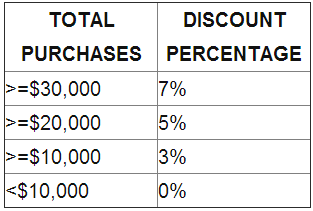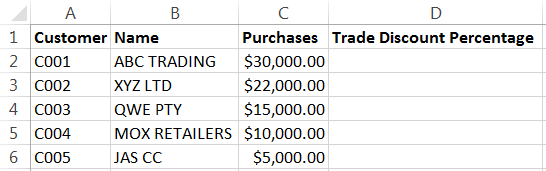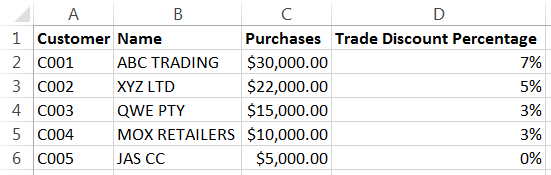# Excel Tip: Calculating Multiple Conditions in a Formula With The Nested IF Statement

Do you need to calculate multiple conditions in a formula? Similar to the example below where a specific trade discount percentage has been used depending on the quantity purchased? If that is the case then you can use the Nested IF statement.

The Nested IF statement enables one to calculate multiple conditions in a formula. As a result more elaborate tests of data can be constructed.

Process: Excel 2003, 2007 and 2010

The If statement can be used to conduct conditional tests on values and formulas. However if more elaborate testing of data will be carried out then the Nested If Statement can be used.

To calculate the trade discount percentage for clients based on the table below;1. Enter the data given below2. Select cell D2 and type the following formula;

=IF(C2>=30000,”7%”,IF(C2>=20000,”5%”,IF(C2>=10000,”3%”,”0%”)))

3. Press enter and copy the formula down

4. The result will be as belowTada! You are now an Excel genius!

For more Excel Tips, subscibe to the Equation Technolioges Blog!

## Need Help Now?